# A simple Python program for an ANN to cover the MNIST dataset – XIII – the impact of regularization

I continue with my growing series on a Multilayer perceptron and the MNIST dataset.

In the last article of the series we made some interesting experiences with the variation of the „leaning rate“. We also saw that a reasonable range for initial weight values should be chosen.

Even more fascinating was, however, the impact of a normalization of the input data on a smooth and fast gradient descent. We drew the conclusion that normalization is of major importance when we use the sigmoid function as the MLP’s activation function – especially for nodes in the first hidden layer and for input data which are on average relatively big. The reason for our concern were saturation effects of the sigmoid functions and other functions with a similar variation with their argument. In the meantime I have tried to make the importance of normalization even more plausible with the help of a a very minimalistic perceptron for which we can analyze saturation effects a bit more in depth; you get to the related article series via the following link:

There we also have a look at other normalizers or feature scalers.

But back to our series on a multi-layer perceptron. You may have have asked yourself in the meantime: Why did he not check the impact of the regularization? Indeed: We kept the parameter Lambda2 for the quadratic regularization term constant in all experiments so far: Lambda2 = 0.2. So, the question about the impact of regularization e.g. on accuracy is a good one.

# How big is the regularization term and how does it evolve during gradient decent training?

I add even one more question: How big is the relative contribution of the regularization term to the total loss or cost function? In our Python program for a MLP model we included a so called quadratic Ridge term:

Lambda2 * 0.5 * SUM[all weights**2], where bias nodes are excluded from the sum.

From various books on Machine Learning [ML] you just learn to choose the factor Lambda2 in the range between 0.01 and 0.1. But how big is the resulting term actually in comparison to the standard cost term, then, and how does the ratio between both terms evolve during gradient descent? What factors influence this ratio?

As we follow a training strategy based on mini-batches the regularization contribution was and is added up to the costs of each mini-batch. So its relative importance varies of course with the size of the mini-batches! Other factors which may also be of some importance – at least during the first epochs – could be the total number of weights in our network and the range of initial weight values.

Regarding the evolution during a converging gradient descent we know already that the total costs go down on the path to a cost minimum – whilst the weight values reach a stable level. So there is a (non-linear!) competition between the regularization term and the real costs of the „Log Loss“ cost function! During convergence the relative importance of the regularization term may therefore become bigger until the ratio to the standard costs reaches an eventual constant level. But how dominant will the regularization term get in the end?

Let us do some experiments with the MNIST dataset again! We fix some common parameters and conditions for our test runs:
As we saw in the last article we should normalize the input data. So, all of our numerical experiments below (with the exception of the last one) are done with standardized input data (using Scikit-Learn’s StandardScaler). In addition initial weights are all set according to the sqrt(nodes)-rule for all layers in the interval [-0.5*sqrt(1/num_nodes), 0.5*sqrt(1/num_nodes)], with num_nodes meaning the number of nodes in a layer. Other parameters, which we keep constant, are:

Parameters: learn_rate = 0.001, decrease_rate = 0.00001, mom_rate = 0.00005, n_size_mini_batch = 500, n_epochs = 800.

I added some statements to the method for cost calculation in order to save the relative part of the regularization terms with respect to the total costs of each mini-batch in a Numpy array and plot the evolution in the end. The changes are so simple that I omit showing the modified code.

# A first look at the evolution of the relative contribution of regularization to the total loss of a mini-batch

How does the outcome of gradient descent look for standardized input data and a Lambda2-value of 0.1?

Lambda2 = 0.1
Results: acc_train: 0.999 , acc_test: 0.9714, convergence after ca. 600 epochs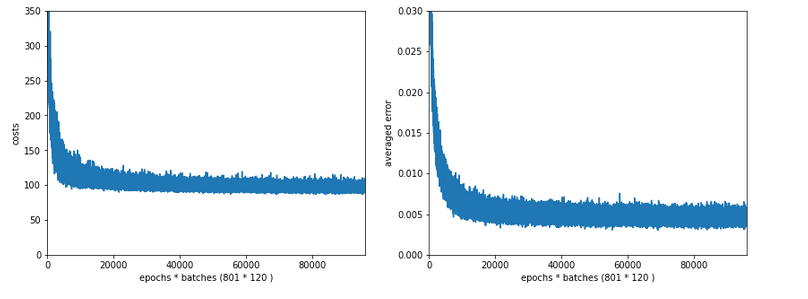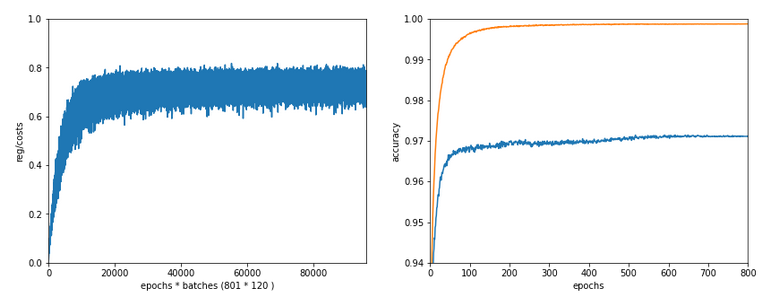We see that the regularization term actually dominates the total loss of a mini-batch at convergence. At least with our present parameter setting. In comparisoin to the total loss of the full training set the contribution is of course much smaller and typically below 1%.

# A small Lambda term

Let us reduce the regularization term via setting Lambda = 0.01. We expect its initial contribution to the costs of a batch to be smaller then, but this does NOT mean that the ratio to the standard costs of the batch automatically shrinks significantly, too:

Lambda2 = 0.01
Results: acc_train: 1.0 , acc_test: 0.9656, convergence after ca. 350 epochs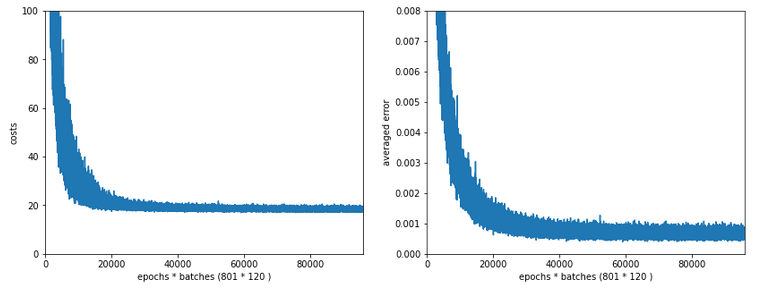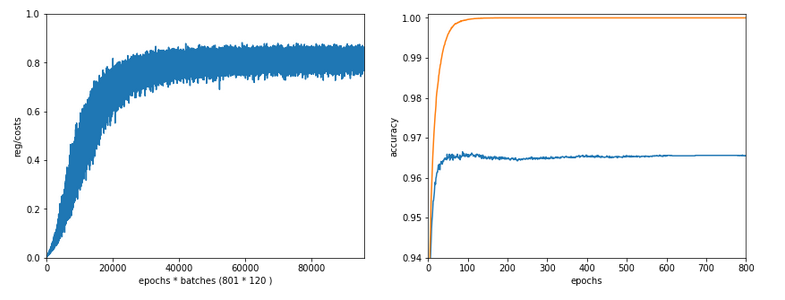Note the absolute scale of the costs in the plots! We ended up at a much lower level of the total loss of a batch! But the relative dominance of regularization at the point of convergence actually increased! However, this did not help us with the accuracy of our MLP-algorithm on the test data set – although we perfectly fit the training set by a 100% accuracy.

In the end this is what regularization is all about. We do not want a total overfitting, a perfect adaption of the grid to the training set. It will not help in the sense of getting a better general accuracy on other input data. A Lambda2 of 0.01 is much too small in our case!

# Slightly bigger regularization with Lambda2 = 0.2

So lets enlarge Lambda2 a bit:
Lambda2 = 0.2
Results: acc_train: 0.9946 , acc_test: 0.9728, convergence after ca. 700 epochs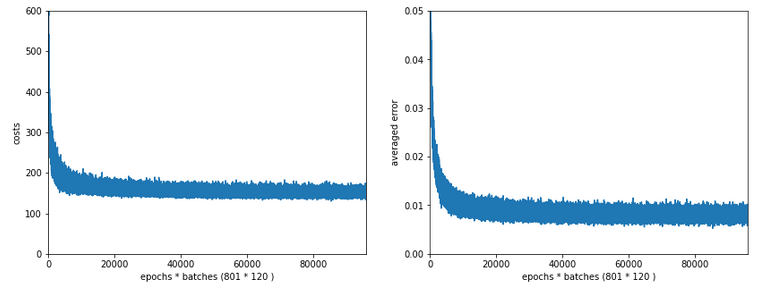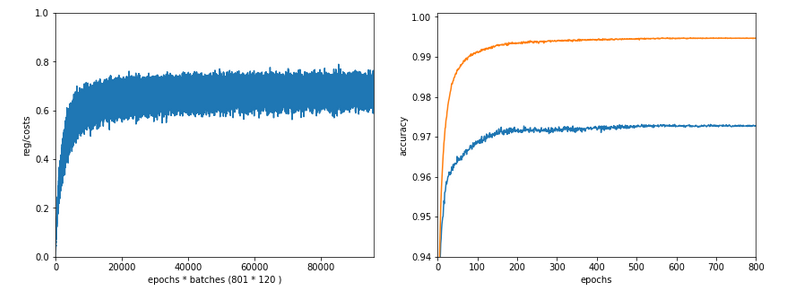We get an improved accuracy!

# Two other cases with significantly bigger Lambda2

Lambda2 = 0.4
Results: acc_train: 0.9858 , acc_test: 0.9693, convergence after ca. 600 epochs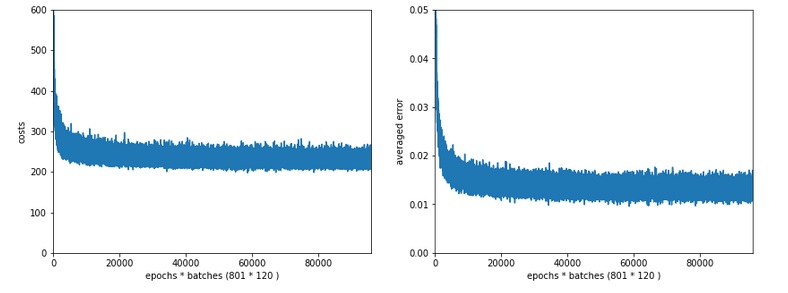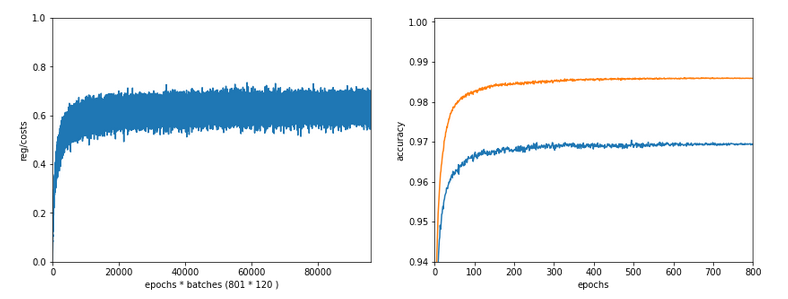Lambda2 = 0.8
Results: acc_train: 0.9705 , acc_test: 0.9588, convergence after ca. 400 epochs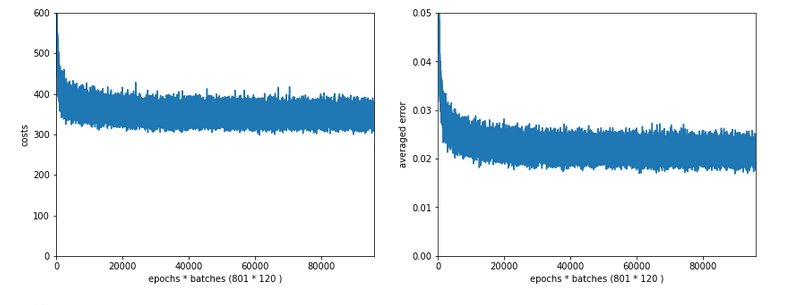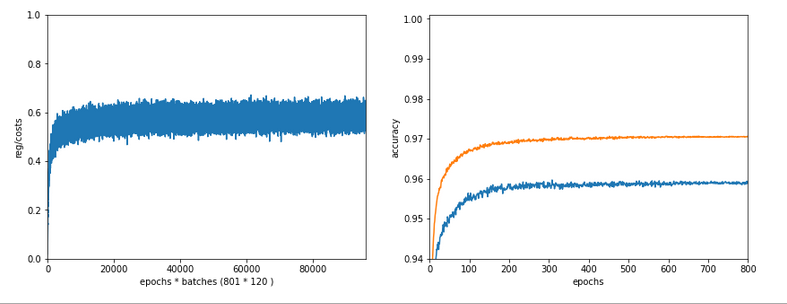OK, but in both cases we see a significant and systematic trend towards reduced accuracy values on the test data set with growing Lambda2-values > 0.2 for our chosen mini-batch size (500 samples).

# Conclusion

We learned a bit about the impact of regularization today. Whatever the exact Lambda2-value – in the end the contribution of a regularization term becomes a significant part of the total loss of a mini-batch when we approached the total cost minimum. However, the factor Lambda2 must be chosen with a reasonable size to get an impact of regularization on the final minimum position in the weight-space! But then it will help to improve accuracy on general input data in comparison to overfitted solutions!

But we also saw that there is some balance to take care of: For an optimum of generalization AND accuracy you should neither make Lambda2 too small nor too big. In our case Lambda2 = 0.2 seems to be a reasonable and good choice. Might be different with other datasets.

All in all studying the impact of a variation of achieved accuracy with the factor for a Ridge regularization term seems to be a good investment of time in ML projects. We shall come back to this point already in the next articles of this series.

In the next article

A simple Python program for an ANN to cover the MNIST dataset – XIV – cluster detection in feature space

we shall start to work on cluster detection in the feature space of the MNIST data before using gradient descent.

# A simple Python program for an ANN to cover the MNIST dataset – V – coding the loss function

We proceed with encoding a Python class for a Multilayer Perceptron [MLP] to be able to study at least one simple examples of an artificial neural network [ANN] in detail. During the articles

we came so far that we could apply the „Feed Forward Propagation“ algorithm [FFPA] to multiple data records of a mini-batch of training data in parallel. We spoke of a so called vectorized form of the FFPA; we used special Linear Algebra matrix operations of Numpy to achieve the parallel operations. In the last article

A simple program for an ANN to cover the Mnist dataset – IV – the concept of a cost or loss function

I commented on the necessity of a so called „loss function“ for the MLP. Although not required for a proper training algorithm we will nevertheless encode a class method to calculate cost values for mini-batches. The behavior of such cost values with training epochs will give us an impression of how good the training algorithm works and whether it actually converges into a minimum of the loss function. As explained in the last article this minimum should correspond to an overall minimum distance of the FFPA results for all training data records from their known correct target values in the result vector space of the MLP.

Before we do the coding for two specific cost or loss functions – namely the „Log Loss„-function and the „MSE„-function, I will briefly point out the difference between standard „*“-operations between multidimensional Numpy arrays and real „dot“-matrix-operations in the sense of Linear Algebra. The latte one follows special rules in multiplying specific elements of both matrices and summing up over the results.

As in all the other articles of this series: This is for beginners; experts will not learn anything new – especially not of the first section.

# Element-wise multiplication between multidimensional Numpy arrays in contrast to the „dot“-operation of linear algebra

I would like to point out some aspects of combining two multidimensional Numpy arrays which may be confusing for Python beginners. At least they were for me 🙂 . As a former physicist I automatically expected a „*“-like operation for two multidimensional arrays to perform a matrix operation in the sense of linear algebra. This lead to problems when I tried to understand Python code of others.

Let us assume we have two 2-dimensional arrays A and B. A and B shall be similar in the sense that their shape is identical, i.e. A.shape = B.shape – e.g (784, 60000):
The two matrices each have the same specific number of elements in their different dimensions.

Whenever we operate with multidimensional Numpy arrays with the same same shape we can use the standard operators „+“, „-„, „*“, „/“. These
operators then are applied between corresponding elements of the matrices. I.e., the mathematical operation is applied between elements with the same position along the different dimensional axes in A and B. We speak of an element-wise operation. See the example below.

This means (A * B) is not equivalent to the C = numpy.dot(A, B) operation – which appears in Linear Algebra; e.g. for vector and operator transformations!

The“dot()“-operation implies a special operation: Let us assume that the shape of A[i,j,v] is

A.shape = (p,q,y)

and the shape of B[k,w,m] is

B.shape = (r,z,s)

with

y = z .

Then in the „dot()“-operation all elements of a dimension „v“ of A[i,j,v] are multiplied with corresponding elements of the dimension „w“ of B[k,w,m] and then the results summed up.

dot(A, B)[i,j,k,m] = sum(A[i,j,:] * B[k,:,m])

The „*“ operation in the formula above is to be interpreted as a standard multiplication of array elements.

In the case of A being a 2-dim array and B being a 1-dimensional vector we just get an operation which could – under certain conditions – be interpreted as a typical vector transformation in a 2-dim vector space.

So, when we define two Numpy arrays there may exist two different methods to deal with array-multiplication: If we have two arrays with the same shape, then the „*“-operation means an element-wise multiplication of the elements of both matrices. In the context of ANNs such an operation may be useful – even if real linear algebra matrix operations dominate the required calculations. The first „*“-operation will, however, not work if the array-shapes deviate.

The „numpy.dot(A, B)„-operation instead requires a correspondence of the last dimension of matrix A with the second to last dimension of matrix B. Ooops – I realize I just used the expression „matrix“ for a multidimensional Numpy array without much thinking. As said: „matrix“ in linear algebra has a connotation of a transformation operator on vectors of a vector space. Is there a difference in Numpy?

Yes, there is, indeed – which may even lead to more confusion: We can apply the function numpy.matrix()

A = numpy.matrix(A),
B = numpy.matrix(B)

then the „*“-operator will get a different meaning – namely that of numpy.dot(A,B):

A * B = numpy.dot(A, B)

So, better read Python code dealing with multidimensional arrays rather carefully ….

To understand this better let us execute the following operations on some simple examples in a Jupyter cell:

```A1 = np.ones((5,3))
A1[:,1] *= 2
A1[:,2] *= 4
print("\nMatrix A1:\n")
print(A1)

A2= np.random.randint(1, 10, 5*3)
A2 = A2.reshape(5,3)
# A2 = A2.reshape(3,5)
print("\n Matrix A2 :\n")
print(A2)

A3 = A1 * A2
print("\n\nA3:\n")
print(A3)

A4 = np.dot(A1, A2.T)
print("\n\nA4:\n")
print(A4)

A5 = np.matrix(A1)
A6 = np.matrix(A2)

A7 = A5 * A6.T
print("\n\nA7:\n")
print(A7)

A8 = A5 * A6
```

We get the following output:

```
Matrix A1:

[[1. 2. 4.]
[1. 2. 4.]
[1. 2. 4.]
[1. 2. 4.]
[1. 2. 4.]]

Matrix A2 :

[[6 8 9]
[9 1 6]
[8 8 9]
[2 8 3]
[5 8 8]]

A3:

[[ 6. 16. 36.]
[ 9.  2. 24.]
[ 8. 16. 36.]
[ 2. 16. 12.]
[
5. 16. 32.]]

A4:

[[58. 35. 60. 30. 53.]
[58. 35. 60. 30. 53.]
[58. 35. 60. 30. 53.]
[58. 35. 60. 30. 53.]
[58. 35. 60. 30. 53.]]

A7:

[[58. 35. 60. 30. 53.]
[58. 35. 60. 30. 53.]
[58. 35. 60. 30. 53.]
[58. 35. 60. 30. 53.]
[58. 35. 60. 30. 53.]]

---------------------------------------------------------------------------
ValueError                                Traceback (most recent call last)
<ipython-input-10-4ea2dbdf6272> in <module>
28 print(A7)
29
---> 30 A8 = A5 * A6
31

/projekte/GIT/ai/ml1/lib/python3.6/site-packages/numpy/matrixlib/defmatrix.py in __mul__(self, other)
218         if isinstance(other, (N.ndarray, list, tuple)) :
219             # This promotes 1-D vectors to row vectors
--> 220             return N.dot(self, asmatrix(other))
221         if isscalar(other) or not hasattr(other, '__rmul__') :
222             return N.dot(self, other)

<__array_function__ internals> in dot(*args, **kwargs)

ValueError: shapes (5,3) and (5,3) not aligned: 3 (dim 1) != 5 (dim 0)

```

This example obviously demonstrates the difference of an multiplication operation on multidimensional arrays and a real matrix „dot“-operation. Note especially how the „*“ operator changed when we calculated A7.

If we instead execute the following code

```A1 = np.ones((5,3))
A1[:,1] *= 2
A1[:,2] *= 4
print("\nMatrix A1:\n")
print(A1)

A2= np.random.randint(1, 10, 5*3)
#A2 = A2.reshape(5,3)
A2 = A2.reshape(3,5)
print("\n Matrix A2 :\n")
print(A2)

A3 = A1 * A2
print("\n\nA3:\n")
print(A3)

```

we directly get an error:

```
Matrix A1:

[[1. 2. 4.]
[1. 2. 4.]
[1. 2. 4.]
[1. 2. 4.]
[1. 2. 4.]]

Matrix A2 :

[[5 8 7 3 8]
[4 4 8 4 5]
[8 1 9 4 8]]

---------------------------------------------------------------------------
ValueError                                Traceback (most recent call last)
<ipython-input-12-c4d3ffb1e683> in <module>
13
14
---> 15 A3 = A1 * A2
16 print("\n\nA3:\n")
17 print(A3)

ValueError: operands could not be broadcast together with shapes (5,3) (3,5)

```

As expected!

# Cost calculation for our ANN

As we want to be able to use different types of cost/loss functions we have to introduce new corresponding parameters in the class’s interface. So we update the „__init__()“-function:

```
def __init__(self,
my_data_set = "mnist",
n_hidden_layers = 1,
ay_nodes_layers = [0, 100, 0], # array which should have as much elements as n_hidden + 2
n_nodes_layer_out = 10,  # expected number of nodes in output layer

my_activation_function = "sigmoid",
my_out_function        = "sigmoid",
my_loss_function       = "LogLoss",

n_size_mini_batch = 50,  # number of data elements in a mini-batch

n_epochs      = 1,
n_max_batches = -1,  # number of mini-batches to use during epochs - > 0 only for testing
# a negative value uses all mini-batches

lambda2_reg = 0.1,     # factor for quadratic regularization term
lambda1_reg = 0.0,     # factor for linear regularization term

vect_mode = 'cols',

figs_x1=12.0, figs_x2=8.0,
legend_loc='upper
right',

b_print_test_data = True

):
'''
Initialization of MyANN
Input:
data_set: type of dataset; so far only the "mnist", "mnist_784" datsets are known
We use this information to prepare the input data and learn about the feature dimension.
This info is used in preparing the size of the input layer.
n_hidden_layers = number of hidden layers => between input layer 0 and output layer n

ay_nodes_layers = [0, 100, 0 ] : We set the number of nodes in input layer_0 and the output_layer to zero
Will be set to real number afterwards by infos from the input dataset.
All other numbers are used for the node numbers of the hidden layers.
n_nodes_out_layer = expected number of nodes in the output layer (is checked);
this number corresponds to the number of categories NC = number of labels to be distinguished

my_activation_function : name of the activation function to use
my_out_function : name of the "activation" function of the last layer which produces the output values
my_loss_function : name of the "cost" or "loss" function used for optimization

n_size_mini_batch : Number of elements/samples in a mini-batch of training data
The number of mini-batches will be calculated from this

n_epochs : number of epochs to calculate during training
n_max_batches : > 0: maximum of mini-batches to use during training
< 0: use all mini-batches

lambda_reg2:    The factor for the quadartic regularization term
lambda_reg1:    The factor for the linear regularization term

vect_mode: Are 1-dim data arrays (vctors) ordered by columns or rows ?

figs_x1=12.0, figs_x2=8.0 : Standard sizing of plots ,
legend_loc='upper right': Position of legends in the plots

b_print_test_data: Boolean variable to control the print out of some tests data

'''

# Array (Python list) of known input data sets
self._input_data_sets = ["mnist", "mnist_784", "mnist_keras"]
self._my_data_set = my_data_set

# X, y, X_train, y_train, X_test, y_test
# will be set by analyze_input_data
# X: Input array (2D) - at present status of MNIST image data, only.
# y: result (=classification data) [digits represent categories in the case of Mnist]
self._X       = None
self._X_train = None
self._X_test  = None
self._y       = None
self._y_train = None
self._y_test  = None

# relevant dimensions
# from input data information;  will be set in handle_input_data()
self._dim_sets     = 0
self._dim_features = 0
self._n_labels     = 0   # number of unique labels - will be extracted from y-data

# Img sizes
self._dim_img      = 0 # should be sqrt(dim_features) - we assume square like images
self._img_h        = 0
self._img_w        = 0

# Layers
# ------
# number of hidden layers
self._n_hidden_layers = n_hidden_layers
# Number of total layers
self._n_total_layers = 2 + self._n_hidden_layers
# Nodes for hidden layers
self._ay_nodes_layers = np.array(ay_nodes_layers)
# Number of nodes in output
layer - will be checked against information from target arrays
self._n_nodes_layer_out = n_nodes_layer_out

# Weights
# --------
# empty List for all weight-matrices for all layer-connections
# Numbering :
# w contains the weight matrix which connects layer 0 (input layer ) to hidden layer 1
# w contains the weight matrix which connects layer 1 (input layer ) to (hidden?) layer 2
self._ay_w = []

# Arrays for encoded output labels - will be set in _encode_all_mnist_labels()
# -------------------------------
self._ay_onehot = None
self._ay_oneval = None

# Known Randomizer methods ( 0: np.random.randint, 1: np.random.uniform )
# ------------------
self.__ay_known_randomizers = [0, 1]

# Types of activation functions and output functions
# ------------------
self.__ay_activation_functions = ["sigmoid"] # later also relu
self.__ay_output_functions     = ["sigmoid"] # later also softmax

# Types of cost functions
# ------------------
self.__ay_loss_functions = ["LogLoss", "MSE" ] # later also othr types of cost/loss functions

# the following dictionaries will be used for indirect function calls
self.__d_activation_funcs = {
'sigmoid': self._sigmoid,
'relu':    self._relu
}
self.__d_output_funcs = {
'sigmoid': self._sigmoid,
'softmax': self._softmax
}
self.__d_loss_funcs = {
'LogLoss': self._loss_LogLoss,
'MSE': self._loss_MSE
}

# The following variables will later be set by _check_and set_activation_and_out_functions()

self._my_act_func  = my_activation_function
self._my_out_func  = my_out_function
self._my_loss_func = my_loss_function
self._act_func = None
self._out_func = None
self._loss_func = None

# list for cost values of mini-batches during training
# The list will later be split into sections for epochs
self._ay_cost_vals = []

# number of data samples in a mini-batch
self._n_size_mini_batch = n_size_mini_batch
self._n_mini_batches = None  # will be determined by _get_number_of_mini_batches()

# number of epochs
self._n_epochs = n_epochs
# maximum number of batches to handle (<0 => all!)
self._n_max_batches = n_max_batches

# regularization parameters
self._lambda2_reg = lambda2_reg
self._lambda1_reg = lambda1_reg

# paramter to allow printing of some test data
self._b_print_test_data = b_print_test_data

# Plot handling
# --------------
# Alternatives to resize plots
# 1: just resize figure  2: resize plus create subplots() [figure + axes]
self._plot_resize_alternative = 1
# Plot-sizing
self._figs_x1 = figs_x1
self._figs_x2 = figs_x2
self._fig = None
self._ax  = None
# alternative 2 does resizing and (!) subplots()
self.initiate_and_resize_plot(self._plot_resize_alternative)

# ***********
# operations
# ***********

# check and handle input data
self._handle_input_data()
# set the ANN structure
self._set_ANN_structure()

# Prepare epoch and batch-handling - sets mini-batch index array, too
self._prepare_epochs_and_batches()

# perform training

start_c = time.perf_counter()
self._fit(b_print=False, b_measure_batch_time=False)
end_c = time.perf_counter()
print('\n\n ------')
print('Total training Time_CPU: ', end_c - start_c)
print("\nStopping program regularily")
sys.exit()
#
```

The way of accessing a method/function by a parameterized „name“-string should already be familiar from other methods. The method with the given name must of course exist in the Python module; otherwise already Eclipse#s PyDev we display errors.

```    '''-- Method to set the loss function--'''
def _check_and_set_loss_function(self):
# check for known loss functions
try:
if (self._my_loss_func not in self.__d_loss_funcs ):
raise ValueError
except ValueError:
print("\nThe requested loss function " + self._my_loss_func + " is not known!" )
sys.exit()

# set the function to variables for indirect addressing
self._loss_func = self.__d_loss_funcs[self._my_loss_func]

if self._b_print_test_data:
z = 2.0
print("\nThe loss function of the ANN/MLP was defined as \""  + self._my_loss_func + '"')
'''
'''
return None
#
```

# The „Log Loss“ function

The „LogLoss“-function has a special form. If „a_i“ characterizes the FFPA result for a special training record and „y_i“ the real known value for this record then we calculate its contribution to the costs as:

Loss = SUM_i [- y_i * log(a_i) – (1 – y_i)*log(1 – a_i)]

This loss function has its justification in statistical considerations – for which we assume that our output function produces a kind of probability distribution. Please see the literature for more information.

Now, due to the encoded result representation over 10 different output dimensions in the MNIST case, corresponding to 10 nodes in the output layer; see the second article of this series, we know that a_i and y_i would be 1-dimensional arrays for each training data record. However, if we vectorize this by treating all records of a mini-batch in parallel we get 2-dim arrays. Actually, we have already calculated the respective arrays in the second to last article.

The rows (1st dim) of a represent the output nodes (training data records, the columns (2nd dim) of a represent the results of the FFPA-result values, which due to our output function have values in the interval ]0.0, 1.0].

The same holds for y – with the difference, that 9 of the values in the rows are 0 and exactly one is 1 for a training record.

The „*“ multiplication thus can be done via a normal element-wise array „multiplication“ on the given 2-dim arrays of our code.

a = ay_ANN_out
y = ay_y_enc

Numpy offers a function „numpy.sum(M)“ for a multidimensional array M, which just sums up all element values. The result is of course a simple scalar.

This information should be enough to understand the following new method:

```    ''' method to calculate the logistic regression loss function '''
def _loss_LogLoss(self, ay_y_enc, ay_ANN_out, b_print = False):
'''
Method which calculates LogReg loss function in a vectorized form on multidimensional Numpy arrays
'''
b_test = False

if b_print:
print("From LogLoss: shape of ay_y_enc =  " + str(ay_y_enc.shape))
print("From LogLoss: shape of ay_ANN_out =  " + str(ay_
ANN_out.shape))
print("LogLoss: ay_y_enc = ", ay_y_enc)
print("LogLoss: ANN_out = \n", ay_ANN_out)
print("LogLoss: log(ay_ANN_out) =  \n", np.log(ay_ANN_out) )

# The following means an element-wise (!) operation between matrices of the same shape!
Log1 = -ay_y_enc * (np.log(ay_ANN_out))
# The following means an element-wise (!) operation between matrices of the same shape!
Log2 = (1 - ay_y_enc) * np.log(1 - ay_ANN_out)

# the next operation calculates the sum over all matrix elements
# - thus getting the total costs for all mini-batch elements
cost = np.sum(Log1 - Log2)

#if b_print and b_test:
# Log1_x = -ay_y_enc.dot((np.log(ay_ANN_out)).T)
# print("From LogLoss: L1 =   " + str(L1))
# print("From LogLoss: L1X =  " + str(L1X))

if b_print:
print("From LogLoss: cost =  " + str(cost))

# The total costs is just a number (scalar)
return cost

```

# The Mean Square Error [MSE] cost function

Although not often used for classification tasks (but more for regression problems) this loss function is so simple that we encode it on the fly. Here we just calculate something like a mean quadratic error:

Loss = 9.5 * SUM_i [ (y_ia_i)**2 ]

This loss function is convex by definition and leads to the following method code:

```    ''' method to calculate the MSE loss function '''
def _loss_MSE(self, ay_y_enc, ay_ANN_out, b_print = False):
'''
Method which calculates LogReg loss function in a vectorized form on multidimensional Numpy arrays
'''
if b_print:
print("From loss_MSE: shape of ay_y_enc =  " + str(ay_y_enc.shape))
print("From loss_MSE: shape of ay_ANN_out =  " + str(ay_ANN_out.shape))
#print("LogReg: ay_y_enc = ", ay_y_enc)
#print("LogReg: ANN_out = \n", ay_ANN_out)
#print("LogReg: log(ay_ANN_out) =  \n", np.log(ay_ANN_out) )

cost = 0.5 * np.sum( np.square( ay_y_enc - ay_ANN_out ) )

if b_print:
print("From loss_MSE: cost =  " + str(cost))

return cost
#
```

# Regularization terms

Regularization is a means against overfitting during training. The trick is that the cost function is enhanced by terms which include sums of linear or quadratic terms of all weights of all layers. This enforces that the weights themselves get minimized, too, in the search for a minimum of the loss function. The less degrees of freedom there are the less the chance of overfitting …

In the literature (see the book hints in the last article) you find 2 methods for regularization – one with quadratic terms of the weights – the so called „Ridge-Regression“ – and one based on a sum of absolute values of the weights – the so called „Lasso regression“. See the books of Geron and Rashka for more information.

Loss = SUM_i [- y_i * log(a_i) – (1 – y_i)*log(1 – a_i)]
+  lambda_2 * SUM_layer [ SUM_nodes [ (w_layer_nodes)**2 ] ]
+  lambda_1 * SUM_layer [ SUM_nodes [ |w_layer_nodes| ] ]

Note that we included already two factors „lambda_2“ and „lamda_1“ by which the regularization terms are multiplied and added to the cost/loss function in the „__init__“-method.

The two related methods are easy to understand:

```    ''' method do calculate the quadratic regularization term for the loss function '''
def _regularize_by_L2(self, b_print=False):

'''
The L2 regularization term sums up all quadratic weights (without the weight for the bias)
over the input and all hidden layers (but not the output layer
The weight for the bias is in the first column (index 0) of the weight matrix -
as the bias node's output is in the first row of the output vector of the layer
'''
ilayer = range(0, self._n_total_layers-1) # this excludes the last layer
L2 = 0.0
for idx in ilayer:
L2 += (np.sum( np.square(self._ay_w[idx][:, 1:])) )
L2 *= 0.5 * self._lambda2_reg
if b_print:
print("\nL2: total L2 = " + str(L2) )
return L2
#

```
```    ''' method do calculate the linear regularization term for the loss function '''
def _regularize_by_L1(self, b_print=False):
'''
The L1 regularization term sums up all weights (without the weight for the bias)
over the input and all hidden layers (but not the output layer
The weight for the bias is in the first column (index 0) of the weight matrix -
as the bias node's output is in the first row of the output vector of the layer
'''
ilayer = range(0, self._n_total_layers-1) # this excludes the last layer
L1 = 0.0
for idx in ilayer:
L1 += (np.sum( self._ay_w[idx][:, 1:]))
L1 *= 0.5 * self._lambda1_reg
if b_print:
print("\nL1: total L1 = " + str(L1))
return L1
#

```

Why do we not start with index „0“ in the weight arrays – self._ay_w[idx][:, 1:]?
The reason is that we do not include the Bias-node in these terms. The weight at the bias nodes of the layers is not varied there during optimization!

Note: Normally we would expect a factor of 1/m, with „m“ being the number of records in a mini-batch, for all the terms discussed above. Such a constant factor does not hamper the principal procedure – if we omit it consistently also for for the regularization terms discussed below. It can be taken care of by choosing smaller „lambda“s and a smaller step size during optimization.

# Inclusion of the loss function calculations within the handling of mini-batches

For our approach with mini-batches (i.e. an approach between pure stochastic and full batch handling) we have to include the cost calculation in our method „_handle_mini_batch()“ to handle mini-batches. Method „_handle_mini_batch()“ is modified accordingly:

```    ''' -- Method to deal with a batch -- '''
def _handle_mini_batch(self, num_batch = 0, b_print_y_vals = False, b_print = False):
'''
For each batch we keep the input data array Z and the output data A (output of activation function!)
for all layers in Python lists
We can use this as input variables in function calls - mutable variables are handled by reference values !
We receive the A and Z data from propagation functions and proceed them to cost and gradient calculation functions

As an initial step we define the Python lists ay_Z_in_layer and ay_A_out_layer
and fill in the first input elements for layer L0
'''
ay_Z_in_layer  = [] # Input vector in layer L0;  result of a matrix operation in L1,...
ay_A_out_layer = [] # Result of activation function

#print("num_batch = " + str(num_batch))
#print("len of ay_mini_batches = " + str(len(self._ay_mini_batches)))
#print("_ay_mini_batches = ")
#print(self._ay_mini_batches[num_batch])

# Step 1: Special treatment of the ANN's input Layer L0
# Layer L0: Fill in the input vector for the ANN'
s input layer L0
ay_idx_batch = self._ay_mini_batches[num_batch]
ay_Z_in_layer.append( self._X_train[ay_idx_batch] ) # numpy arrays can be indexed by an array of integers
#print("\nPropagation : Shape of X_in = ay_Z_in_layer = " + str(ay_Z_in_layer.shape))
if b_print_y_vals:
print("\n idx, expected y_value of Layer L0-input :")
for idx in self._ay_mini_batches[num_batch]:
print(str(idx) + ', ' + str(self._y_train[idx]) )

# Step 2: Layer L0: We need to transpose the data of the input layer
ay_Z_in_0T       = ay_Z_in_layer.T
ay_Z_in_layer = ay_Z_in_0T

# Step 3: Call the forward propagation method for the mini-batch data samples
self._fw_propagation(ay_Z_in = ay_Z_in_layer, ay_A_out = ay_A_out_layer, b_print = b_print)

if b_print:
# index range of layers
ilayer = range(0, self._n_total_layers)
print("\n ---- ")
print("\nAfter propagation through all " + str(self._n_total_layers) + " layers: ")
for il in ilayer:
print("Shape of Z_in of layer L" + str(il) + " = " + str(ay_Z_in_layer[il].shape))
print("Shape of A_out of layer L" + str(il) + " = " + str(ay_A_out_layer[il].shape))

# Step 4: To be done: cost calculation for the batch
ay_y_enc = self._ay_onehot[:, ay_idx_batch]
ay_ANN_out = ay_A_out_layer[self._n_total_layers-1]
# print("Shape of ay_ANN_out = " + str(ay_ANN_out.shape))

total_costs_batch = self._calculate_loss_for_batch(ay_y_enc, ay_ANN_out, b_print = False)
self._ay_cost_vals.append(total_costs_batch)

# Step 5: To be done: gradient calculation via back propagation of errors
# Step 6: Adjustment of weights

# try to accelerate garbage handling
if len(ay_Z_in_layer) > 0:
del ay_Z_in_layer
if len(ay_A_out_layer) > 0:
del ay_A_out_layer

return None
#
```

Note that we save the cost values of every batch in the 1-dim array „self._ay_cost_vals“. This array can later on easily be split into arrays for epochs.

The whole process must be supplemented by a method which does the real cost value calculation:

```    ''' -- Main Method to calculate costs -- '''
def _calculate_loss_for_batch(self, ay_y_enc, ay_ANN_out, b_print = False, b_print_details = False ):
'''
Method which calculates the costs including regularization terms
The cost function is called according to an input parameter of the class
'''
pure_costs_batch = self._loss_func(ay_y_enc, ay_ANN_out, b_print = False)

if ( b_print and b_print_details ):
print("Calc_Costs: Shape of ay_ANN_out = " + str(ay_ANN_out.shape))
print("Calc_Costs: Shape of ay_y_enc = " + str(ay_y_enc.shape))
if b_print:
print("From Calc_Costs: pure costs of a batch =  " + str(pure_costs_batch))

# Add regularitzation terms - L1: linear reg. term, L2: quadratic reg. term
# the sums over the weights (squared) have to be performed for each batch again due to intermediate corrections
L1_cost_contrib = 0.0
L2_cost_contrib = 0.0
if self._lambda1_reg > 0:
L1_cost_contrib = self._regularize_by_L1( b_print=False )
if self._lambda2_reg > 0:
L2_cost_contrib = self._regularize_by_L2( b_print=False )

total_costs_batch = pure_costs_batch + L1_cost_contrib + L2_cost_contrib
#
```

# Conclusion

By the steps
discussed above we completed the inclusion of a cost value calculation in our class for every step dealing with a mini-batch during training. All cost values are saved in a Python list for later evaluation. The list can later be split with respect to epochs.

In contrast to the FFP-algorithm all array-operations required in this step were simple element-wise operations and summations over all array-elements.

Cost value calculation obviously is simple and pretty fast regarding CPU-consumption! Just test it yourself!

In the next article we shall analyze the mathematics behind the calculation of the partial derivatives of our cost-function with respect to the many weights at all nodes of the different layers. We shall see that the gradient calculation reduces to remarkable simple formulas describing a kind of back-propagation of the error terms [y_ia_i] through the network.

We will not be surprised that we need to involve some real matrix operations again as in the FFPA !

# A simple Python program for an ANN to cover the MNIST dataset – I – a starting point

For beginners both in Python and Machine Learning [ML] the threshold to do some real programming and create your own Artificial Neural Network [ANN] seems to be relatively high. Well, some readers might say: Why program an ANN by yourself at a basic Python level at all when Keras and TensorFlow [TF] are available? Answer: For learning! And eventually to be able to do some things TF has not been made for. And as readers of this blog will see in the future, I have some ideas along this line …

So I thought, just let me set up a small Python3 and Numpy based program to create a simple kind of ANN – a „Multilayer Perceptron“ [MLP] – and train it for the MNIST dataset. I expected that my readers and I myself would earn something on various methods used in ML during our numerical experiments. Well, we shall see ..-

Regarding ANN-theory I take a brutal shortcut and assume that my readers are already acquainted with the following topics:

1. what simple neural networks with „hidden“ layers look like and what its weights are,
2. what a „cost function“ is and how the gradient descent method works,
3. what logistic regression is and what the cost function J for it looks like,
4. why we need the back propagation of deviations from known values and why it gives you the required partial derivatives of a typical cost function with respect to an ANN’s „weights“,
5. what a mini-batch approach to weight optimization is.

I cannot spare you the effort of studying most of these topics in advance. Otherwise I would have to write an introductory book on ML myself. [I would do, but you need to give me a sponsor 🙂 ] But even if you are not fully acquainted with all the named topics: I shall briefly comment on each and every of the points in the forthcoming articles. The real basics are, however, much better and more precisely documented in the literature; e.g. in the books of Geron and Rashka (see the references at the end of this article). I recommend to read one of these books whilst we move on with a sequence of steps to build the basic code for our MLP.

We need a relatively well defined first objective for the usage of our ANN. We shall concentrate on classification tasks. As a first example we shall use the conventional MNIST data set. The MNIST data set consists of images of handwritten numbers with 28×28 pixels [px]. It is a standard data set used in many elementary courses on ML. The challenge for our ANN is that it should be able to recognize hand-written digits from a digitized gray-color image after some training.

Note that this task does NOT require the use of a fully fletched multi-layer MLP. „Stochastic Gradient Descent“-approaches for a pure binary classificator to determine (linear) separation surfaces in combination with a „One-versus-All“ strategy for multi-category-classification may be sufficient. See chapters 3 to 5 in the book of Geron for more information.

Regarding the build up of the ANN program, I basically follow an approach described by S. Raschka in his book (see the second to last section for a reference). However, at multiple points I take my freedom to organize the code differently and comment in my own way … I am only a beginner in Python; I hope my insights are helpful for others in the same situation.

In any case you should make yourself familiar with numpy arrays and their „shapes“. I assume that you understand the multidimensional structure of Numpy arrays ….

# Wording

To avoid confusion, I use the following wording and synonyms:

Category: Each input data element is associated with a category to which it belongs. In our case a category
corresponds to a „digit“. The classification algorithm (here: the MLP) may achieve an ability to predict the association of an unknown MNIST like input data sample with its correct category. It should – after some training – detect the (non-linear) separation interfaces for categories in a multidimensional feature space. In the case of MNIST we speak about ten categories corresponding to 10 digits, including zero.

Label: A category may be described by a label. Training data may provide a so called „target label array“ _y_train for all input data. We must be prepared to transform target labels for input data into a usable form for an ANN, i.e. into a vectorized form, which selects a specific category out of many. This process is called „label encoding“.

Input data set: A complete „set“ of input data. Such a set consists of individual „elements“ or „records„. Another term which we I shall frequently use for such an element is a „sample„. The MNIST input set of training data consists of 60000 records or samples – which we provide via an array _X_train. The array is two dimensional as each sample consists of values more multiple properties.

Feature: A sample of the input data set may be equivalent to a mathematical vector, whose elements specify (numerical) values for multiple properties – so called „features“ – of a sample. Thus, input samples correspond to points in a multidimensional feature space.

Output data set: A complete set of output data after a so called „propagation“ through the ANN for the input data set. „Propagation“means a series of defined mathematical transformations of the original features data of the input sample. The number of samples or records in the output data sets is equal to the number of records in the input data set. The output set will be represented by a Numpy array „_ay_ANN_out„.

A data record or sample of the input data set: One distinct element of the input data set (and its array). Note that such an element itself may be a multidimensional array covering all features in a distinct form. Such arrays represents a so called „tensor“.

A data record of the output data set: One distinct element of the output data set. Note that such an element itself may be an array covering all possible categories in a distinct form. E.g., we may be given a „probability“ for each category – which allows us to decide with which of the categories we should associate the output element.

# A simple MLP network – layers, nodes, weights

An AN network is composed of a series of horizontally and/or vertically arranged layers with nodes. The nodes represent the artificial neurons. A MLP is an ANN which has a rather simple structure: It consists of an input layer, multiple sequential intermediate „hidden“ layers, and an output layer. All nodes of a specific layer are connected with all nodes of neighboring (!) layers, only. We speak of a „dense“ or fully „connected layer“ structure.

The simplifying sketch below displays an ANN with just three sequentially arranged layers – an input layer, a „hidden“ middle layer and an output layer.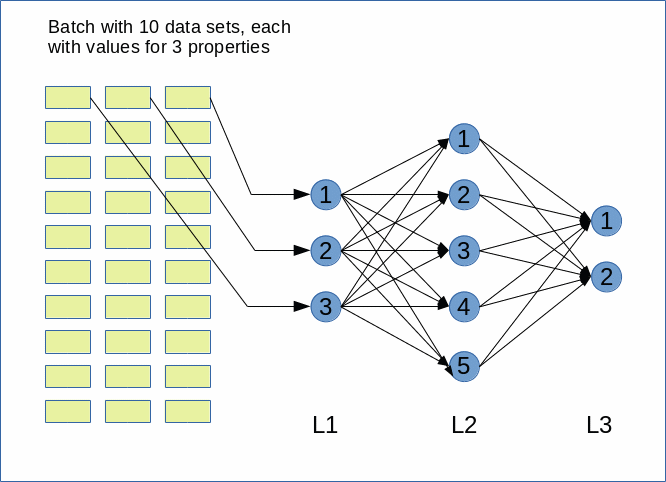Note that in general there can be (many) more hidden layers than just one. Note also that modern ANNs (e.g. Convoluted Networks) may have a much more complicated topological structure with hundreds of layers. There may also be
cascaded networks where a specific layer has connections to many more than just the neighbor layers.

Input layer and its number of nodes
To feed input data into the MLP we need an „input layer“ with sufficient input nodes. How many? Well, this depends on the number of features your data set represents. In the MNIST case a sample image contains 28×28 pixels. For each pixel with a gray value (integer number between 0 and 256) given. So a typical image represents 28×28 = 768 different „features“ – i.e. 786 numbers for „gray“-values between 0 and 255. We need as many input nodes in our MLP to represent the full image information by the input layer.

For other input data the number of features may be different; in addition features my follow a multidimensional order or organization which first must be „flattened“ out in one dimension. The number of input nodes must then be adjusted accordingly. The number of input nodes should, therefore, be a parameter or be derived from information on the type of input data. The way of how you map complicated and structured features to input layers and whether you map all data to a one dimensional input vector is a question one should think about carefully. (Most people today treat e.g. a time dimension of input data as just a special form of a feature – I regard this as questionable in some cases, but this is beyond this article series …)

For our MLP we always assume a mapping of features to a flat one dimensional vector like structure.

Output layer and its number of nodes
We shall use our MLP for classification tasks in the beginning. We, therefore, assume that the output of the ANN should allow for the distinction between „strong>NC“ different categories an input data set can belong to. In case of the MNIST dataset we can distinguish between 10 different digits. Thus an output layer must in this case comprise 10 different nodes. To be able to cover other data sets with a different number of categories the number of output nodes must be a parameter of our program, too.

How we indicate the association of a (transformed) sample at the output layer to a category numerically – by a probability number between „0“ and „1“ or just a „1“ at the right category and zeros otherwise – can be a matter of discussion. It is also a question of the cost function we wish to use. We will come back to this point in later articles.

The numbers of „hidden layers“ and their nodes
We want the numbers of nodes on „hidden layers“ to be parameters for our program. For simple data as MNIST images we do not need big networks, but we want to be able to play around a bit with 1 up to 3 layers. (For an ANN to recognize hand written MNIST digits an input layer „L0“ and only one hidden layer „L1“ before an output layer „L2“ are fully sufficient. Nevertheless in most of our experiments we will actually use 2 hidden layers. There are three reasons: You can approximate any continuous function with two hidden layers (with a special non-linear activation function; see below) and an output layer (with just a linear output function). The other reason is that the full mathematical complexity of „learning“ of a MLP appears with two hidden layers (see a later article).

Activation and output functions
The nodes in hidden layers use a so called „activation function“ to transform aggregated input from different feeding nodes of the previous layer into one distinct value within a defined interval – e.g. between -1 and 1. Again, we should be prepared to have a program parameter to choose between different „activation functions“.

We should be aware of the fact that the nodes of the output layers need special consideration as the „activation function“ there
produces the final output – which in turn must allow for a distinction of categories. This may lead to a special form – e.g. a kind of probability function. So, the type of the „output function“ should also be regarded as variable parameter.

# A Python class for our ANN and its interface

I develop my code as a Python module in an Eclipse/PyDev IDE, which itself uses a virtual Python3 environment. I described the setup of such a development environment in detail in another previous article of this blog. In the resulting directory structure of the PyDev project I place a module „myann.py“ at the location „…../ml_1/mynotebooks/mycode/myann.py“. This file shall contain the code of class „MyANN“ for our ANN.

## Modules and libraries to import

We need to import some libraries at the head of our Python program first:

```'''
Module to create a simple layered neural network for the MNIST data set
Created on 23.08.2019
@author: ramoe
'''
import numpy as np
import math
import sys
import time
import tensorflow
from sklearn.datasets import fetch_mldata
from sklearn.datasets import fetch_openml
from keras.datasets import mnist as kmnist
from scipy.special import expit
from matplotlib import pyplot as plt
#from matplotlib.colors import ListedColormap
#import matplotlib.patches as mpat
#from keras.activations import relu

```

Why do I import „tensorflow“ and „keras“?
Well, only for the purpose to create the input data of MNIST quickly. Sklearn’s „fetchml_data“ is doomed to end. The alternative „fetch_openml“ does not use caching in some older versions and is also in general terribly slow. But, „keras“, which in turn needs tensorflow as a backend, provides its own tool to provide the MNIST data.

We need „scipy“ to get an optimized version of the so called „sigmoid„-function – which is an important version of an activation function. We shall use it most of the time. „numpy“ and „math“ are required for fast array- and math-operations. „time“ is required to measure the run time of program segments and „mathplotlib“ will help us to visualize some information gathered during and after training.

# The „__init__“-function of our class MyANN

We encapsulate most of the required functionality in a class and its methods. Python provides the „__init__“-function, which we can use as a kind of „constructor“ – although it technically is not the same as a constructor in other languages. Anyway, we can use it as an interface to feed in parameters and to initialize variables of a class instance.

We shall build up our „__init__()“-function during the next articles step by step. In the beginning we shall only focus on attributes and methods of our class required to import the MNIST data and put them into Numpy arrays and to create the basic network layers.

Parameters

```class MyANN:
def __init__(self,
my_data_set = "mnist",
n_hidden_layers = 1,
ay_nodes_layers = [0, 100, 0], # array which should have as much elements as n_hidden + 2
n_nodes_layer_out = 10,  # number of nodes in output layer

my_activation_function = "sigmoid",
my_out_function        = "sigmoid",

vect_mode = 'cols',

figs_x1=12.0, figs_x2=8.0,
legend_loc='upper right'
)
:
'''
Initialization of MyANN
Input:
data_set: type of dataset; so far only the "mnist", "mnist_784" and the "mnist_keras" datsets are known.
We use this information to prepare the input data and learn about the feature dimension.
This info is used in preparing the size of the input layer.
n_hidden_layers = number of hidden layers => between input layer 0 and output layer n
ay_nodes_layers = [0, 100, 0 ] : We set the number of nodes in input layer_0 and the output_layer to zero
Will be set to real number afterwards by infos from the input dataset.
All other numbers are used for the node numbers of the hidden layers.
n_nodes_layer_out = expected number of nodes in the output layer (is checked);
this number corresponds to the number of categories to be distinguished

my_activation_function : name of the activation function to use
my_out_function : name of the "activation" function of the last layer whcih produces the output values

vect_mode: Are 1-dim data arrays (vectors) ordered by columns or rows ?

figs_x1=12.0, figs_x2=8.0 : Standard sizing of plots ,
legend_loc='upper right': Position of legends in the plots

'''
```

You see that I defined multiple parameters, which are explained in the Python „doc“-string. We use a „string“ to choose the dataset to train our ANN on. To be able to work on other data sets later on we assume that specific methods for importing a variety of special input data sets are implemented in our class. This requires that the class knows exactly which kinds of data sets it is capable to handle. We provide an list with this information below. The other parameters should be clear from their inline documentation.

# Initialization of class attributes

We first initialize a bunch of class attributes which we shall use to define the network of layers, nodes, weights, to keep our input data and functions.

```        # Array (Python list) of known input data sets
self.__input_data_sets = ["mnist", "mnist_784", "mnist_keras"]
self._my_data_set = my_data_set

# X, y, X_train, y_train, X_test, y_test
# will be set by analyze_input_data
# X: Input array (2D) - at present status of MNIST image data, only.
# y: result (=classification data) [digits represent categories in the case of Mnist]
self._X       = None
self._X_train = None
self._X_test  = None
self._y       = None
self._y_train = None
self._y_test  = None

# relevant dimensions
# from input data information;  will be set in handle_input_data()
self._dim_sets     = 0
self._dim_features = 0
self._n_labels     = 0   # number of unique labels - will be extracted from y-data

# Img sizes
self._dim_img      = 0 # should be sqrt(dim_features) - we assume square like images
self._img_h        = 0
self._img_w        = 0

# Layers
# ------
# number of hidden layers
self._n_hidden_layers = n_hidden_layers
# Number of total layers
self._n_total_layers = 2 + self._n_hidden_layers
# Nodes for hidden layers
self._ay_nodes_layers = np.array(ay_nodes_layers)
# Number of nodes in output layer - will be checked against information from
target arrays
self._n_nodes_layer_out = n_nodes_layer_out

# Weights
# --------
# empty List for all weight-matrices for all layer-connections
# Numbering :
# w contains the weight matrix which connects layer 0 (input layer ) to hidden layer 1
# w contains the weight matrix which connects layer 1 (input layer ) to (hidden?) layer 2
self._ay_w = []

# Known Randomizer methods ( 0: np.random.randint, 1: np.random.uniform )
# ------------------
self.__ay_known_randomizers = [0, 1]

# Types of activation functions and output functions
# ------------------
self.__ay_activation_functions = ["sigmoid"] # later also relu
self.__ay_output_functions     = ["sigmoid"] # later also softmax

# the following dictionaries will be used for indirect function calls
self.__d_activation_funcs = {
'sigmoid': self._sigmoid,
'relu':    self._relu

}
self.__d_output_funcs = {
'sigmoid': self._sigmoid,
'softmax': self._softmax
}

# The following variables will later be set by _check_and set_activation_and_out_functions()
self._my_act_func = my_activation_function
self._my_out_func = my_out_function
self._act_func = None
self._out_func = None

# Plot handling
# --------------
# Alternatives to resize plots
# 1: just resize figure  2: resize plus create subplots() [figure + axes]
self._plot_resize_alternative = 1
# Plot-sizing
self._figs_x1 = figs_x1
self._figs_x2 = figs_x2
self._fig = None
self._ax  = None
# alternative 2 does resizing and (!) subplots()
self.initiate_and_resize_plot(self._plot_resize_alternative)

# ***********
# operations
# ***********
# check and handle input data
self._handle_input_data()

print("\nStopping program regularily")
sys.exit()

```

To make things not more complicated as necessary I omit the usage of „properties“ and a full encapsulation of private attributes. For convenience reasons I use only one underscore for some attributes and functions/methods to allow for external usage. This is helpful in a testing phase. However, many items can in the end be switched to really private properties or methods.

# List of known input datasets

The list of known input data sets is kept in the variable „self.__input_data_sets“. The variables

self._X, self._X_train, self._X_test, self._y, self._y_train, self._y_test

will be used to keep all sample data of the chosen dataset – i.e. the training data, the test data for checking of the reliability of the algorithm after training and the corresponding target data (y_…) for classification – in distinct array variables during code execution. The target data in the MNIST case contain the digit a specific sample image ( of _X_train or _X_test..) represents.

All of the named attributes will become Numpy arrays. A method called „_handle_input_data(self)“ will load the (MNIST) input data and fill the arrays.

The input arrays „X_…“ will via their dimensions provide the information on the number of data sets (_dim_sets) and the number of features (_dim_features). Numpy provides the various dimensions of multidimensional arrays in form of a tuple.

The target data arrays „_y_…“ provides the number of „categories“ (MNIST: 10 digits) the ANN must distinguish after training. We keep this number in the
attribute „_n_labels“. It is also useful to keep the pixel dimensions of input image data. At least for MNIST we assume quadratic images (_img_h = img_w = _dim_img).

# Layers and weights

The number of total layers („_n_total_layers“) is by 2 bigger than the number of hidden layers (_n_hidden_layers).

We take the number of nodes in the layers from the respective list provided as an input parameter „ay_nodes_layers“ to our class. We transform the list into a numpy array „_ay_nodes_layers“. The expected number of nodes in the output layer is used for consistency checks and saved in the variable „_n_nodes_layer_out“.

The „weights“ of an ANN must be given in form of matrices: A weight describes a connection between two nodes of different adjacent layers. So we have as many connections as there are node combinations (nodex_(N+1), nodey_N), with „nodex_N“ meaning a node on layer L_N and nodey_(N+1) a node on layer L_(N+1).

As the number of layers is not fixed, but can be set by the user, I use a Python list „_ay_w“ to collect such matrices in the order of layer_0 (input) to layer_n (output).

Weights, i.e. the matrix elements, must initially be set as random numbers. To provide such numbers we have to use randomizer functions. Depending on the kind (floating point numbers, integer numbers) of random numbers to produce we use at least two randomizers (randint, uniform). For the weights we use the uniform-randomizer.

Allowed activation and output function names are listed in Python dictionaries which point to respective methods. This allows for an „indirect addressing“ of these functions later on. You may recognize this by the direct reference of the dictionary elements to defined class methods (no strings are used!).

For the time being we work with the „sigmoid“ and the „relu“ functions for activation and the „sigmoid“ and „softmax“ functions for output creation. The attributes „self._act_func“ and „self._out_func“ are used later on to invoke the functions requested by the respective parameters of the classes interface.

The final part of the code segment given above is used for plot-sizing with the help of „matplotlib“; a method „initiate_and_resize_plot()“ takes care of this. It can use 2 alternative ways of doing so.

# Read and provide the input data

Now let us turn to some methods. We first need to read in and prepare the input data. We use a method „_handle_input_data()“ to work on this problem. For the time being we have only three different ways to load the MNIST dataset from different origins.

```    # Method to handle different types of input data sets
def _handle_input_data(self):
'''
Method to deal with the input data:
- check if we have a known data set ("mnist" so far)
- reshape as required
- analyze dimensions and extract the feature dimension(s)
'''
# check for known dataset
try:
if (self._my_data_set not in self._input_data_sets ):
raise ValueError
except ValueError:
print("The requested input data" + self._my_data_set + " is not known!" )
sys.exit()

# handle the mnist original dataset
if ( self._my_data_set == "mnist"):
mnist = fetch_mldata('MNIST original')
self._X, self._y = mnist["data"], mnist["target"]
print("Input data for dataset " + self._my_data_set + " : \n" + "Original shape of X = " + str(self._X.shape) +
"\n" + "Original shape of y = " + str(self._y.shape))
self._X_train, self._X_test, self._y_train, self._y_test = self._X[:60000], self._X[60000:],
self._y[:60000], self._y[60000:]

# handle the mnist_784 dataset
if ( self._my_data_set == "mnist_784"):
mnist2 = fetch_openml('mnist_784', version=1, cache=True, data_home='~/scikit_learn_data')
self._X, self._y = mnist2["data"], mnist2["target"]
print ("data fetched")
# the target categories are given as strings not integers
self._y = np.array([int(i) for i in self._y])
print ("data modified")
print("Input data for dataset " + self._my_data_set + " : \n" + "Original shape of X = " + str(self._X.shape) +
"\n" + "Original shape of y = " + str(self._y.shape))
self._X_train, self._X_test, self._y_train, self._y_test = self._X[:60000], self._X[60000:], self._y[:60000], self._y[60000:]

# handle the mnist_keras dataset
if ( self._my_data_set == "mnist_keras"):
(self._X_train, self._y_train), (self._X_test, self._y_test) = kmnist.load_data()
len_train =  self._X_train.shape
#print(len_train)
print("Input data for dataset " + self._my_data_set + " : \n" + "Original shape of X_train = " + str(self._X_train.shape) +
"\n" + "Original Shape of y_train = " + str(self._y_train.shape))
len_test =  self._X_test.shape
#print(len_test)
print("Original shape of X_test = " + str(self._X_test.shape) +
"\n" + "Original Shape of y_test = " + str(self._y_test.shape))
self._X_train = self._X_train.reshape(len_train, 28*28)
self._X_test  = self._X_test.reshape(len_test, 28*28)

# Common Mnist handling
if ( self._my_data_set == "mnist" or self._my_data_set == "mnist_784" or self._my_data_set == "mnist_keras" ):
self._common_handling_of_mnist()

# Other input data sets can not yet be handled

```

We first check, whether the input parameter fits a known dataset – and raise an error if otherwise. The data come in different forms for the three sources of MNIST. For each set we want to extract the arrays

self._X_train, self._X_test, self._y_train, self._y_test.

We have to do this a bit differently for the 3 cases. Note that the „mnist_784“ set from „fetch_openml“ gives the target category values in form of strings and not integers. We correct this directly after loading.

The fastest method for importing the MNIST dataset is based on „keras“; the keras function „kmnist.load_data()“ provides already a 60000:10000 ratio for training and test data. However, we get the images in a (60000, 28, 28) array shape; we therefore reshape the „_X_train“-array to (60000, 784) and „_X_test“-array to (10000, 784).

# Analysis of the input data and the one-hot-encoding of the target labels

A further handling of the MNIST data requires some common analysis.

```    # Method for common input data handling of Mnist data sets
def _common_handling_of_mnist(self):
print("\nFinal input data for dataset " + self._my_data_set +
" : \n" + "Shape of X_train = " + str(self._X_train.shape) +
"\n" + "Shape of y_train = " + str(self._y_train.shape) +
"\n" + "Shape of X_test = " + str(self._X_test.shape) +
"\n" + "Shape of y_test = " + str(self._y_test.shape)
)

# mixing the training indices
shuffled_index = np.random.permutation(60000)
self._X_train, self._y_train = self._X_train[shuffled_index], self._y_train[shuffled_index]

# set
dimensions
self._dim_sets = self._y_train.shape
self._dim_features = self._X_train.shape
self._dim_img = math.sqrt(self._dim_features)
# we assume square images
self._img_h = int(self._dim_img)
self._img_w = int(self._dim_img)

# Print dimensions
print("\nWe have " + str(self._dim_sets) + " data sets for training")
print("Feature dimension is " + str(self._dim_features) + " (= " + str(self._img_w)+ "x" + str(self._img_h) + ")")

# we need to encode the digit labels of mnist
self._get_num_labels()
self._encode_all_mnist_labels()
```

As you see we retrieve some of our class attributes which we shall use during training and do some printing. This is trivial. Not as trivial is, however, the handling of the output data:

What shape do we expect for the „_X_train“ and „_y_train“? Each element of the input data set is an array with values for all features. Thus the „_X_train.shape“ should be (60000, 784). For _y_train we expect a simple integer describing the digit to which the MNIST input image corresponds. Thus we expect a one dimensional array with _y_train.shape = (60000). So far, so good …

But: The output data of our ANN for one input element will be provided as an array of values for our 10 different categories – and not as a simple number. To account for this we need to encode the „_y_train“-data, i.e. the target labels, into an usable array form. We use two methods to achieve this:

```    # Method to get the number of target labels
def _get_num_labels(self):
self._n_labels = len(np.unique(self._y_train))
print("The number of labels is " + str(self._n_labels))

# Method to encode all mnist labels
def _encode_all_mnist_labels(self, b_print=True):
'''
We shall use vectorized input and output - i.e. we process a whole batch of input data sets in parallel
(see article in the Linux blog)
The output array will then have the form OUT(i_out_node, idx) where
i_out_node enumerates the node of the last layer (i.e. the category)
idx enumerates the data set within a batch,
After training, if y_train[idx] = 6, we would expect an output value of OUT[6,idx] = 1.0 and OUT[i_node, idx]=0.0 otherwise
for a categorization decision in the ideal case. Realistically, we will get a distribution of numbers over the nodes
with values between 0.0 and 1.0 - with hopefully the maximum value at the right node OUT[6,idx].

The following method creates an arrays OneHot[i_out_node, idx] with
OneHot[i_node_out, idx] = 1.0, if i_node_out =  int(y[idx])
OneHot(i_node_out, idx] = 0.0, if i_node_out != int(y[idx])

This will allow for a vectorized comparison of calculated values and knwon values during training
'''

self._ay_onehot = np.zeros((self._n_labels, self._y_train.shape))
# ay_oneval is just for convenience and printing purposes
self._ay_oneval = np.zeros((self._n_labels, self._y_train.shape, 2))

if b_print:
print("\nShape of y_train = " + str(self._y_train.shape))
print("Shape of ay_onehot = " + str(self._ay_onehot.shape))

# the next block is just for illustration purposes and a better understanding
if b_print:
values = enumerate(self._y_train[0:12])
print("\nValues of the enumerate structure for the first 12 elements : = ")

for iv in values:
print(iv)

# here we prepare the array for vectorized
comparison
print("\nLabels for the first 12 datasets:")
for idx, val in enumerate(self._y_train):
self._ay_onehot[val, idx ]   = 1.0
self._ay_oneval[val, idx, 0] = 1.0
self._ay_oneval[val, idx, 1] = val

if b_print:
print("\nShape of ay_onehot = " + str(self._ay_onehot.shape))
print(self._ay_onehot[:, 0:12])
#print("Shape of ay_oneval = " + str(self._ay_oneval.shape))
#print(self._ay_oneval[:, 0:12, :])

```

The first method only determines the number of labels (= number of categories). We see from the code of the second method that we encode the target labels in the form of two arrays. The relevant one for our optimization algorithm will be „_ay_onehot“. This array is 2-dimensional. Why?

# Working with mini-batches

A big advantage of the weight optimization method we shall use later on during training of our MLP is that we will perform weight adjustment for a whole bunch of training samples in one step. Meaning:

We propagate a whole bunch of test data samples in parallel through the grid to get an array with result data (output array) for all samples.

Such a bunch is called a „batch“ and if it is significantly smaller than the whole set of training data – a „mini-batch„.

Working with „mini-batches“ during the training and learning phase of an ANN is a compromise between

• using the full data set (for gradient evaluation) during each training step („batch approach„)
• and using just one sample of the training data set for gradient descent and weight corrections („stochastic approach„).

See chapter 4 of the book of Geron and chapter 2 in the book of Raschka for some thorough information on this topic.

The advantage of mini-batches is that we can use vectorized linear algebra operations over all elements of the batch. Linear Algebra libraries are optimized to perform the resulting vector and matrix operations on modern CPUs and GPUs.

You really should keep the following point in mind to understand the code for the propagation and optimization algorithms discussed in forthcoming articles:
The so called „cost function“ will be determined as some peculiar sum over all elements of a batch and the usual evaluation of partial derivatives during gradient descent will be based on matrix operations involving all input elements of a defined batch!

Mini-batches also will help during training in so far as we look at a bunch of multiple selected samples in parallel to achieve bigger steps of our gradient guided descent into an minimum of the cost hyperplane in the beginning – with the disadvantage of making some jumpy stochastic turns on the cost hyperplane instead of a smoother approach.

I probably lost you now 🙂 . The simpler version is: Keep in mind that we later on will work with batches of training data samples in parallel!

However, the separation interface for our categories in the feature space must in the end be adjusted with respect to all given data points of the training set. This means we must perform the training successively for a whole sequence of mini-batches which together cover all available training samples.

What is the shape of the output array?
A single element of the batch is an array of 784 feature values. The corresponding output array is an array with values for 10 categories (here digits). But, what about a whole bunch of test data, i.e. a „batch“?

As I have explained already in my last article
Numpy matrix multiplication for layers of simple feed forward ANNs
the output array for a batch of test data will have the form „_ay_a_Out[i_out_node, idx]“ with:

• i_out_node enumerating the node of the last layer, i.e. a possible category
• idx enumerating the data sample within a batch of training data

We shall construct the output function such that it provides something like „probability“ values within the interval [0,1] for each node of the output layer. We define a perfectly working MLP as one which – after training – produces a „1.0“ at the correct category node (i.e. the expected digit) and „0.0“ at all other output nodes.

One-hot-encoding of labels
Later on we must compare the real results for the training samples with the expected correct values. To be able to do this we must build up a 2-dim array of the same shape as „_ay_a_out“ with correct output values for all test samples of the batch. E.g.: If we expect the digit 7 for the input array of a sample with index idx within the set of training data, we need a 2-dim output array with the element [[0,0,0,0,0,0,0,1,0,0], idx]. The derivation of such an array from a given category label is called „one-hot-encoding„.

By using Numpy’s zero()-function and Pythons „enumerate()“-function we can achieve such an encoding for all data elements of the training data set. See the method „_encode_all_mnist_labels()“. Thus, the array „_ay_onehot“ will have a shape of (10, 60000). From this 2-dim array we can later slice out bunches of consecutive test data for mini-batches. The array „_ay_oneval“ is provided for convenience and print purposes, only: it provides the expected digit value in addition.

# First tests via a Jupyter notebook

Let us test the import of the input data and the label encoding with a Jupyter notebook. In previous articles I have described already how to use such a notebook. I set up a Jupyter notebook called „myANN“ (in my present working directory „/projekte/GIT/ai/ml1/mynotebooks“).

I start it with

```myself@mytux:/projekte/GIT/ai/ml1> source bin/activate
(ml1) myself@mytux:/projekte/GIT/ai/ml1> jupyter notebook
[I 15:07:38.754 NotebookApp] jupyter_tensorboard extension loaded.
[I 15:07:38.754 NotebookApp] Serving notebooks from local directory: /projekte/GIT/ai/ml1
[I 15:07:38.754 NotebookApp] The Jupyter Notebook is running at:
[I 15:07:38.754 NotebookApp] http://localhost:8888/?token=06c2626c8724f65d1e3c4a50457da0d6db414f88a40c7baf
[I 15:07:38.755 NotebookApp] Use Control-C to stop this server and shut down all kernels (twice to skip confirmation).
[C 15:07:38.771 NotebookApp]
```

and add two cells. The first one is for the import of libraries.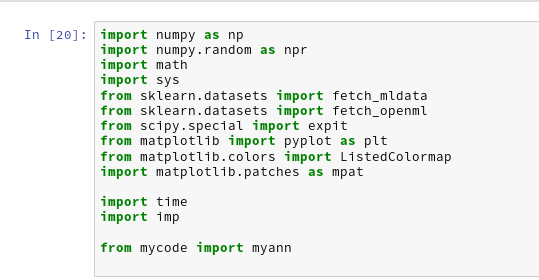By the last line I import my present class code. With the second cell I create an instance of my class; the „__init__()“-function is automatically executed ad calls the other methods defined so far: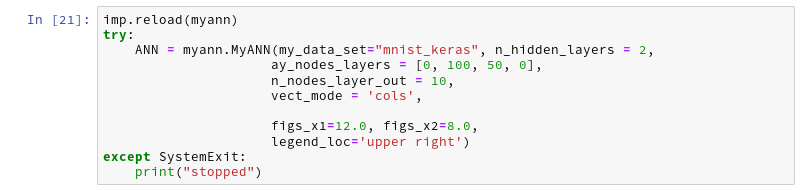Note that the display of „_ay_onehot“ shows the categories in vertical (!) direction (rows) and the index for the input data element in horizontal direction (columns)! You see that the labels in the enumerate structure correspond to the „1“s in the „_ay_onehot“-array.

# Conclusion

Importing the MNIST dataset into Numpy arrays via Keras is simple – and has a good performance. We have learned a bit about „one-hot-encoding“ and prepared an array „_ay_onehot“, which we shall use during ANN training and weight optimization. It will allow us to calculate a difference between the actual output values of the ANN at the nodes of the output layer and a „1.0“ value at the node for the expected sample category and „0.0“ otherwise.

In the next article
A simple program for an ANN to cover the Mnist dataset – II
we shall define initial weights for our ANN.